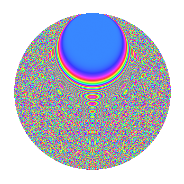# Properties

 Label 349.2.iLevel 349 Weight 2 Character orbit i Rep. character $$\chi_{349}(9,\cdot)$$ Character field $$\Q(\zeta_{87})$$ Dimension 1568 Newforms 1 Sturm bound 58 Trace bound 0

# Related objects

## Defining parameters

 Level: $$N$$ = $$349$$ Weight: $$k$$ = $$2$$ Character orbit: $$[\chi]$$ = 349.i (of order $$87$$ and degree $$56$$) Character conductor: $$\operatorname{cond}(\chi)$$ = $$349$$ Character field: $$\Q(\zeta_{87})$$ Newforms: $$1$$ Sturm bound: $$58$$ Trace bound: $$0$$

## Dimensions

The following table gives the dimensions of various subspaces of $$M_{2}(349, [\chi])$$.

Total New Old
Modular forms 1680 1680 0
Cusp forms 1568 1568 0
Eisenstein series 112 112 0

## Trace form

 $$1568q - 58q^{2} - 58q^{3} - 28q^{4} - 62q^{5} - 72q^{6} - 60q^{7} + 23q^{8} - 30q^{9} + O(q^{10})$$ $$1568q - 58q^{2} - 58q^{3} - 28q^{4} - 62q^{5} - 72q^{6} - 60q^{7} + 23q^{8} - 30q^{9} - 56q^{10} - 56q^{11} - 69q^{12} - 56q^{13} - 60q^{14} - 67q^{15} - 24q^{16} - 58q^{17} + 40q^{18} - 169q^{19} - 72q^{20} + 46q^{21} - 51q^{22} - 47q^{23} + q^{24} - 52q^{25} + 11q^{26} - 28q^{27} - 6q^{28} - 66q^{29} - 37q^{30} - 10q^{31} - 52q^{32} - 70q^{33} - 44q^{34} - 42q^{35} - 124q^{36} - 159q^{37} - 118q^{38} - 32q^{39} - 82q^{40} - 403q^{42} - 35q^{43} + 78q^{44} - 76q^{45} - 63q^{46} - 32q^{47} + 22q^{48} - 32q^{49} + 308q^{50} + 44q^{51} + 255q^{52} - 46q^{53} - 51q^{54} + 48q^{55} - 39q^{56} - 83q^{57} - 46q^{58} - 42q^{59} - 742q^{60} + 190q^{61} - 31q^{62} - 89q^{63} + 149q^{64} - 130q^{65} + 385q^{66} - 24q^{67} - q^{68} + 48q^{69} - 6q^{70} - 48q^{71} + 243q^{72} - 81q^{73} + 75q^{74} - 32q^{75} - 67q^{76} - 48q^{77} - 547q^{78} - 106q^{79} + 148q^{80} + 418q^{81} - 50q^{82} - 304q^{83} + 338q^{84} + 90q^{85} - 46q^{86} + 102q^{87} - 116q^{88} + 340q^{89} - 126q^{90} - 112q^{91} - 106q^{92} - q^{93} - 91q^{94} + 120q^{95} - 914q^{96} - 58q^{97} + 316q^{98} - 131q^{99} + O(q^{100})$$

## Decomposition of $$S_{2}^{\mathrm{new}}(349, [\chi])$$ into irreducible Hecke orbits

Label Dim. $$A$$ Field CM Traces $q$-expansion
$$a_2$$ $$a_3$$ $$a_5$$ $$a_7$$
349.2.i.a $$1568$$ $$2.787$$ None $$-58$$ $$-58$$ $$-62$$ $$-60$$